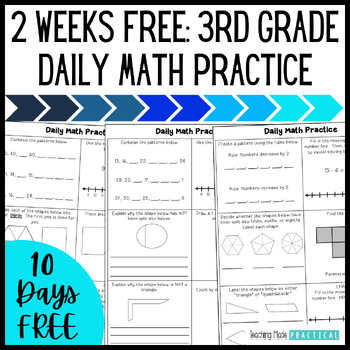# 3rd Grade Daily Math Practice / Math Morning Work: 1 Month FREE3rd, Homeschool
Subjects
Standards
Resource Type
Formats Included
• PDF
Pages
10 pages

### Description

This no prep 3rd grade FREEBIE makes preparing math morning work or daily math practice easy. The math skills are scaffolded, starting easier and getting progressively more difficult as the quarter progresses. Supports are in place throughout the morning work to help students be able to complete the morning work / math review both independently and successfully.

Save over 30% when you buy 3rd Grade morning work / math practice for all year.

20 days (1 month) of daily math practice is included in this free resource. Every day, students will answer a math question from each of the 5 Common Core math domains for 3rd grade, as well as a challenge question.

Students will practice the following Common Core math standards throughout the quarter:

Operations and Algebraic Thinking: 3.OA.D.9

Identify arithmetic patterns (including patterns in the addition table or multiplication table), and explain them using properties of operations.

Number and Operations in Base 10: 3.NBT.A.2

Fluently add and subtract within 1000 using strategies and algorithms based on place value, properties of operations, and/or the relationship between addition and subtraction. (The focus in this resource is building place value skills using a number line.)

Number and Operations - Fractions: 3.NF.A.1

Understand a fraction 1/b as the quantity formed by 1 part when a whole is partitioned into b equal parts; understand a fraction a/b as the quantity formed by a parts of size 1/b.

Measurement and Data: 3.MD.C.6, 3.MD.D.8

Solve real world and mathematical problems involving perimeters of polygons, including finding the perimeter given the side lengths, finding an unknown side length, and exhibiting rectangles with the same perimeter and different areas or with the same area and different perimeters.

Geometry: 3.G.A.1

Understand that shapes in different categories (e.g., rhombuses, rectangles, and others) may share attributes (e.g., having four sides), and that the shared attributes can define a larger category (e.g., quadrilaterals). Recognize rhombuses, rectangles, and squares as examples of quadrilaterals, and draw examples of quadrilaterals that do not belong to any of these subcategories.

This free resource can be used for Grade 3 math morning work, daily math practice, or math homework.

Save over 30% when you buy 3rd Grade morning work for the entire year:

Or, buy the morning work by quarter.

3rd Grade Morning Work: Quarter 1

3rd Grade Morning Work: Quarter 2

3rd Grade Morning Work: Quarter 3

3rd Grade Morning Work: Quarter 4

Total Pages
10 pages
Included
Teaching Duration
N/A
Report this Resource to TpT
Reported resources will be reviewed by our team. Report this resource to let us know if this resource violates TpT’s content guidelines.

### Standards

to see state-specific standards (only available in the US).
Understand that shapes in different categories (e.g., rhombuses, rectangles, and others) may share attributes (e.g., having four sides), and that the shared attributes can define a larger category (e.g., quadrilaterals). Recognize rhombuses, rectangles, and squares as examples of quadrilaterals, and draw examples of quadrilaterals that do not belong to any of these subcategories.
Understand a fraction 1/𝘣 as the quantity formed by 1 part when a whole is partitioned into 𝘣 equal parts; understand a fraction 𝘢/𝑏 as the quantity formed by 𝘢 parts of size 1/𝘣.
Identify arithmetic patterns (including patterns in the addition table or multiplication table), and explain them using properties of operations. For example, observe that 4 times a number is always even, and explain why 4 times a number can be decomposed into two equal addends.
Fluently add and subtract within 1000 using strategies and algorithms based on place value, properties of operations, and/or the relationship between addition and subtraction.
Solve real world and mathematical problems involving perimeters of polygons, including finding the perimeter given the side lengths, finding an unknown side length, and exhibiting rectangles with the same perimeter and different areas or with the same area and different perimeters.